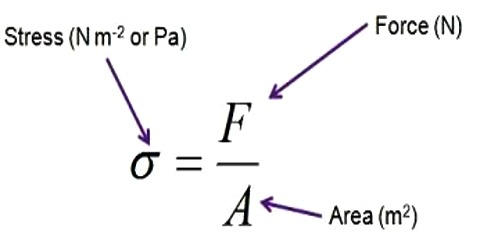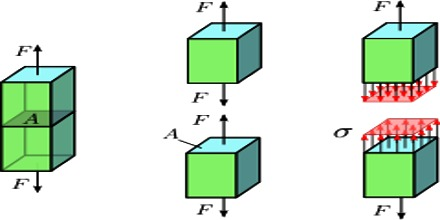Physics

# Stress DefinitionStress: If an external force is applied on an object, then according to Newton’s third law of motion a reaction force is developed in the object due to elasticity. The magnitudes of these action and reaction forces are equal and opposite to each other. Hence the magnitude of the action or reaction force per unit area of the object is called stress.

In a simple language, stress is the force per unit area on an object. When some external forces are applied to a body, then the body offers internal resistance to these forces. The stress applied to a material is the force per unit area applied to the material. The maximum stress a material can stand before it breaks is called the breaking stress or ultimate tensile stress.Let the area of the object = A and applied force = F

Stress = Force / Ares = F/A

Types of stresses: There are three types of stresses. viz.,

(1) Longitudinal stress, (2) Shearing stress and (3) Volume stress

Unit of stress: In the M.K.S. and S.I. methods the absolute unit of stress is Newton/m2, in short Nm-2.

Dimension of Stress = Stress = Force / Ares = (Mass x Acceleration) / (length)2

[MLT-2/L-2] = [ML-1T-2]